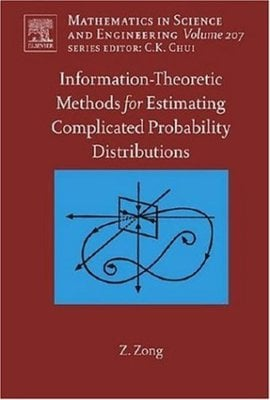Hurry, item low in stock!

# Information-theoretic methods for estimating complicated probability distributions - Zhi ZongHurry only 1 in stock!
FREE Delivery on ALL Orders!
Title
Information-theoretic methods for estimating complicated probability distributions
Author
Zhi Zong
format
Hardback
Publisher
Elsevier
Language
English
UK Publication Date
20060815

Mixing up various disciplines frequently produces something that are profound and far-reaching. Cybernetics is such an often-quoted example. Mix of information theory, statistics and computing technology proves to be very useful, which leads to the recent development of information-theory based methods for estimating complicated probability distributions.
Estimating probability distribution of a random variable is the fundamental task for quite some fields besides statistics, such as reliability, probabilistic risk analysis (PSA), machine learning, pattern recognization, image processing, neural networks and quality control. Simple distribution forms such as Gaussian, exponential or Weibull distributions are often employed to represent the distributions of the random variables under consideration, as we are taught in universities. In engineering, physical and social science applications, however, the distributions of many random variables or random vectors are so complicated that they do not fit the simple distribution forms at al.
Exact estimation of the probability distribution of a random variable is very important. Take stock market prediction for example. Gaussian distribution is often used to model the fluctuations of stock prices. If such fluctuations are not normally distributed, and we use the normal distribution to represent them, how could we expect our prediction of stock market is correct? Another case well exemplifying the necessity of exact estimation of probability distributions is reliability engineering. Failure of exact estimation of the probability distributions under consideration may lead to disastrous designs.
There have been constant efforts to find appropriate methods to determine complicated distributions based on random samples, but this topic has never been systematically discussed in detail in a book or monograph. The present book is intended to fill the gap and documents the latest research in this subject.
Determining a complicated distribution is not simply a multiple of the workload we use to determine a simple distribution, but it turns out to be a much harder task. Two important mathematical tools, function approximation and information theory, that are beyond traditional mathematical statistics, are often used. Several methods constructed based on the two mathematical tools for distribution estimation are detailed in this book. These methods have been applied by the author for several years to many cases. They are superior in the following senses:
(1) No prior information of the distribution form to be determined is necessary. It can be determined automatically from the sample;
(2) The sample size may be large or small;
(3) They are particularly suitable for computers.

It is the rapid development of computing technology that makes it possible for fast estimation of complicated distributions.

The methods provided herein well demonstrate the significant cross influences between information theory and statistics, and showcase the fallacies of traditional statistics that, however, can be overcome by information theory.

Key Features:

- Density functions automatically determined from samples
- Free of assuming density forms
- Computation-effective methods suitable for PC

##### We are Rated Excellent on TrustpilotHere's what you say about us...
Type
BOOK
Keyword Index
Distribution (Probability theory)|Information theory.|Approximation theory.
Country of Publication
Netherlands
Number of Pages
299